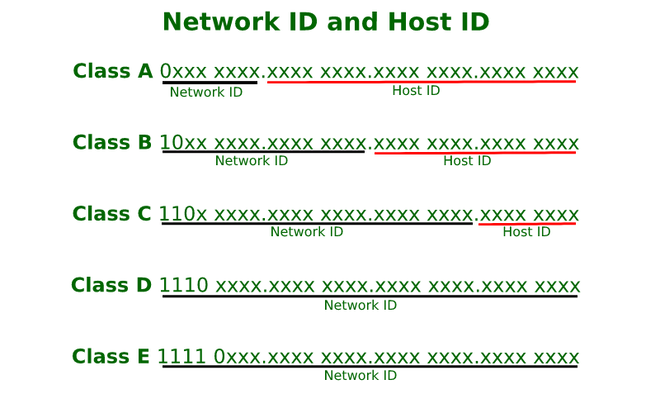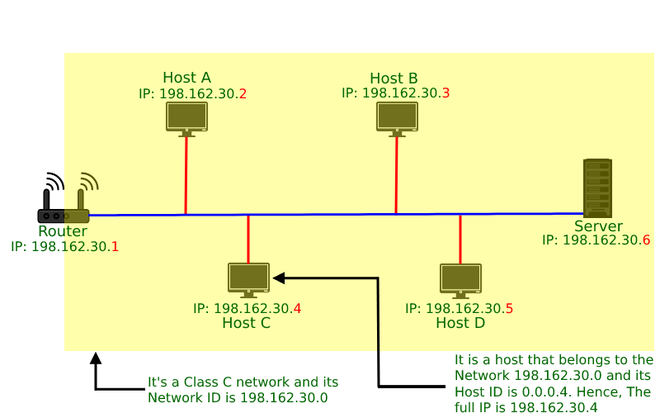# What is “network ID” and “host ID” in IP Addresses?

• Last Updated : 06 Jun, 2022

IP addresses are divided into 5 classes namely, Class A, Class B, Class C, Class D, and Class E. This concept came in around the 1980s. Where

• Class A is generally used for big networks such as the ISP networks.
• Class B is used for medium to large networks like some big organizations.
• Class C addresses are generally used for smaller networks.
• Class D addresses are used for Multicasting.
• Class E addresses are reserved addresses and they are used for experimental purposes.

Range of All Classes:

```Class A: 1.0.0.0 to 127.255.255.255
Class B: 128.0.0.0 to 191.255.255.255
Class C: 192.0.0.0 to 223.255.255.255
Class D: 224.0.0.0 to 239.255.255.255
Class E: 240.0.0.0 to 255.255.255.255```

Network ID:

A network ID or NetID is the fragment of IP address that classifies the network for a specified host i.e., it tells us which network the host belongs to, generally comprised of one to up to four octets in dotted-decimal representation.

In dotted-decimal representation, an IP address is divided into four octets, and based on which class the IP address belongs to its octets are further divided into network ID and HOST ID.

For Class A first octet represents network ID as the prefix of the first octet is 0, it uses the remaining 7 bits for network ID, for Class B first and second octets represent network ID the prefix for class B is 10 so it uses the remaining 14 bits for network ID, for Class C first, second and third octet represents network ID the prefix of class C is 110 so it uses the remaining 21 bits for network ID, Class D, and Class E are reserved.Host ID:

It is the fragment of an IP address that uniquely classifies a host on a specified TCP/IP network. A host ID can be found simply by ANDing the IP address in binary form with its respective default subnet mask (in binary form). The other fragment of an IP address is the network ID, which identifies the network to which the host belongs.Host ID and Network ID

For Example, In the above Image, the  IP address of host C is 198.162.30.4 which means it belongs to class C, so its default subnet mask will be 255.255.255.0; Now applying AND on both, it will give the host ID 0.0.0.4 and network ID 198.162.30.0.

Question 1: What is the network address if the destination address is 200.45.34.56 and the subnet mask is 255.255.255.240?

Solution: So, from the given question we see that this address belongs to class C.

To find the network address we perform AND operation between the given two inputs

200.45.34.00111000 AND 255.255.255.11110000 [converting the last octet into binary form]

we get 200.45.34.48 as a  network address.

Question 2: A company is granted the site address 181.56.0.0. The company needs 1000 subnets. Find the subnet mask

Solution:  The given address belongs to class B

and the default subnet mask of class B is, 255.255.0.0

to get 1000 subnets we perform a log operation

log 1000=10

So, we require extra 10 bits from 255.255.00000000.00000000 to get the subnet mask i.e.;  255.255.00000000.000000000

So, the required subnet mask is 255.255.11111111.11000000 or 255.255.255.192

My Personal Notes arrow_drop_up
Recommended Articles
Page :